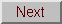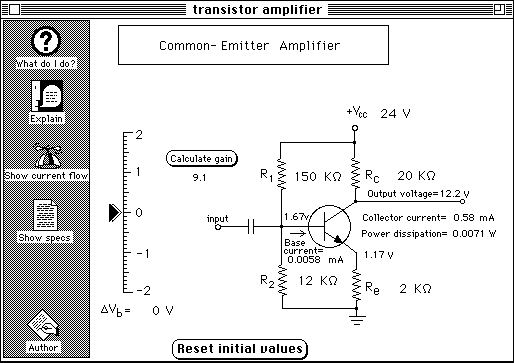## Common Emitter AC Amplifier

The purpose of this circuit is to amplify a small AC input signal, such as an audio or radio frequency signal. A small AC voltage is applied to the input, through a coupling capacitor. The output voltage (on the collector terminal of the transistor) will have an AC component that is an amplified version of the input signal, superimposed on a DC voltage. The ratio of the AC component of the output to the AC component of the input is called the "gain". The gain is usually between 5 an 20. Sometimes two or more such circuits are connected in series to obtain higher total gain; a coupling capacitor must be used between the stages to pass only the AC component of the signal to the next stage and to prevent the DC component of the collector voltage of one stage from upsetting the operation of the next stage. (Hence, such a circuit is useful only as an AC amplifier; to amplify DC signals you should use an operational amplifier circuit).

You can simulate a low-frequency AC input manually by rocking the input slider back and forth about zero and observing how much the collector (output) voltage changes. Can you see that the changes in the output voltage are greater than the changes in the input voltage? This is the "gain". (The gain is determined roughly be the ratio of the collector resistor, Rc, to the emitter resistor, Re).

You can automate the calculation of the AC gain by pressing the "Calculate gain" button; this changes the base voltage by 0.1 volt, measures the resulting collector voltage change, and calculates the gain from that.

To see the current flow through the transistor, click on the "Show current flow" button.

The DC component of the base voltage is set by the voltage divider composed of R1 and R2. This is called the base "bias" voltage. Setting the base bias voltage sets the DC component of the emitter voltage, the collector current, and the collector voltage. The best bias setting is to make the DC component of the collector voltage about one-half of Vcc, which allows the largest output AC voltage swing. The maximum possible output AC voltage will then be about Vcc volts peak-to-peak. The amplifier only works well if the output peak-to-peak AC voltage is smaller than Vcc volts peak-to-peak. The output waveform will be "clipped" if you attempt to obtain a higher output voltage.

You can change the bias resistors R1 and R2, the collector resistor, Rc, the emitter resistor, Re, and the power supply voltage, Vcc.

You can also inspect and modify the specifications of the transistor - click on the "Show specs" button on the left; this display a table of several specifications (such as the transistor's current gain, beta) that you can modify.

### Student Questions

1. Launch the Hypercard stack "Transistor amplifier".

2. Inspect the screen layout. Click on the "What do I do?" button and the "Explain" buttons and read the pop-up help fields.

3. Adjust the bias resistors R1 and R2 so that the collector voltage is about 1/2 of the supply voltage Vcc .

4. To simulate an AC input voltage, move the slider about zero and observe the changes in the output voltage at the collector. Do you see evidence of amplification? The gain of the circuit is defined as deltaVout/deltaVin; compute this ratio and compare it to the ratio of Rc to Re.

5. Click on the "Show specs" button to display the table of transistor specifications. Change the beta to a value twice as great and determine how this change effects the operation of the circuit. Is the gain of the circuit effected by the beta? (You can use the short-cut method of measuring the gain by clicking on the "Calculate gain" button, which changes the input voltage by 0.1 volts and records the resulting change in output (collector) voltage).

Is the bias point (collector voltage with no input signal) changes by increasing the beta?

Is anything in this circuit effected by the beta? Explain.

What happens of the beta is too low? (return the beta to its previous value before going on).

6. The gain of the circuit is controlled by Rc and Re. Try making the gain equal to 20. Did the change in Rc and Re change the bias point? You should re-adjust the bias resistors to make the collector voltage about 1/2 of the supply voltage Vcc .

7. Does Vcc have any effect on the gain? If you change the value of Vcc , is it necessary to re-adjust the bias point?

8. Did you find that this computer simulation was helpful in any way in furthering your understanding of this circuit? Why or why not?